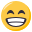# Easiest way to get a random float within a range?

• Hey sorry for the potentially dumb question. I know Math.randInt() and Math.random() exist, but what's the best way to get a random float with say 2 decimal points within a range?

So far I have

``````randomFloat = Math.randInt(1, 8) - Math.range(Math.random(), 0.0, 0.7);
``````

Which works but how do I shave down the decimal points to something more end-user friendly?

• Okay, found Engine.doubleToString• Okay now how do I convert the string back to a float so I can use it to control a moduleEdit: ok adding another Math function to the string converts it backeasy